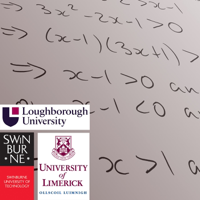FEATURED PODCAST 👇# MathsCasts

• ## Calculating a Fourier series (MathsCasts)

18:39 | Mar 7th, 2017

Deals with the details of calculating Fourier series coefficients for a function.

• ## Why are circles, ellipses, parabolas and hyperbolas called 'conic sections'? Part 2 (MathsCasts)

11:25 | Mar 7th, 2017

We solve simultaneously the equations of a plane and a cone and show that the intersections are circles, parabolas, ellipses, hyperbolas, straight lines or just the origin.

• ## Introduction to half range (even or odd expansion) Fourier series (MathsCasts)

04:47 | Mar 7th, 2017

Introduces the idea of half-range Fourier series and addresses the question of why we use them.

• ## How do odd or even functions make calculation of Fourier series simpler? (MathsCasts)

03:48 | Mar 7th, 2017

Demonstrates that symmetry of functions helps us to shorten the calculation of the integrals needed for the Fourier coefficients.

• ## What is a Laplace transform and why do you need to study it? (MathsCasts)

04:11 | Mar 6th, 2017

Brief introduction to why we use Laplace transforms, with mention of tables.

• ## The first shift theorem (MathsCasts)

02:09 | Mar 6th, 2017

Brief and simple introduction to the first shift theorem for Laplace transforms.